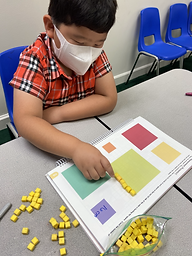Ms. Joan

Target 1​

Lesson Type:

New

Measurment

:

Techniques and Formulas

Explore the concept of perimeter using blocks.

1:

Understand that perimeter is a measurement of the outside length of a 2D shape.

Kindergarden

Vocabulary:

Perimeter, Measure

Activities:

• With the use of the centimeter blocks, students measure the different sizes of squares
• Using a centimeter squares, students measure one side of different sizes of triangle.Home Exploration

Guiding Questions:Absent Students:

Target 2

:

1:

Understand that area is a measurement of how much space a 2D object is taking up.

2:

Estimate the space a figure is occupying.

Kindergarden

Vocabulary:

Area, estimate

Activities:

• using blocks, students measure rectangles with different lengths.
• Students measure uneven 2D shapes.
• Students estimate uneven shapes before they get the actual measurement using blocks.Home Exploration

Guiding Questions:Target 3

:

Vocabulary:

Activities:Home Exploration